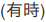# Scalable Data Science

### Course Project by Akinwande Atanda

The html source url of this databricks notebook and its recorded Uji:#Tweet Analytics

## Creating Machine Learning Pipeline without Loop

• The elasticNetParam coefficient is fixed at 1.0
• Read the Spark ML documentation for Logistic Regression
• The dataset “pos_neg_category” can be split into two or three categories as done in the next note. In this note, the dataset is randomly split into training and testing data
• This notebook can be upload to create a job for scheduled training and testing of the logistic classifier algorithm

#### Import the required python libraries:

• From PySpark Machine Learning module import the following packages:
• Pipeline;
• binarizer, tokenizer and hash tags from feature package;
• logistic regression from regression package;
• Multi class evaluator from evaluation package
• Read the PySpark ML package documentation for more details
``````
from pyspark.ml import *
from pyspark.ml import Pipeline
from pyspark.ml.feature import *
from pyspark.ml.classification import *
from pyspark.ml.tuning import *
from pyspark.ml.evaluation import *
from pyspark.ml.regression import *

``````

#### Set the Stages (Binarizer, Tokenizer, Hash Text Features, and Logistic Regression Classifier Model)

``````
bin = Binarizer(inputCol = "category", outputCol = "label", threshold = 0.5) # Positive reviews > 0.5 threshold
tok = Tokenizer(inputCol = "review", outputCol = "word") #Note: The column "words" in the original table can also contain sentences that can be tokenized
hashTF = HashingTF(inputCol = tok.getOutputCol(), numFeatures = 50000, outputCol = "features")
lr = LogisticRegression(maxIter = 10, regParam = 0.0001, elasticNetParam = 1.0)
pipeline = Pipeline(stages = [bin, tok, hashTF, lr])

``````

#### Convert the imported featurized dataset to dataframe

``````
df = table("pos_neg_category")

``````

#### Randomly split the dataframe into training and testing set

``````
(trainingData, testData) = df.randomSplit([0.7, 0.3])

``````

#### Fit the training dataset into the pipeline

``````
model = pipeline.fit(trainingData)

``````

#### Test the predictability of the fitted algorithm with test dataset

``````
predictionModel=model.transform(testData)

``````
``````
display(predictionModel.select("label","prediction", "review", "probability")) # Prob of being 0 (negative) against 1 (positive)

``````
``````
predictionModel.select("label","prediction", "review", "probability").show(10) # Prob of being 0 (negative) against 1 (positive)

``````

#### Assess the accuracy of the algorithm

``````
evaluator = MulticlassClassificationEvaluator(
labelCol="label", predictionCol="prediction", metricName="precision")
accuracy = evaluator.evaluate(predictionModel)

print("Logistic Regression Classifier Accuracy Rate = %g " % (accuracy))
print("Test Error = %g " % (1.0 - accuracy))

``````

Updated: